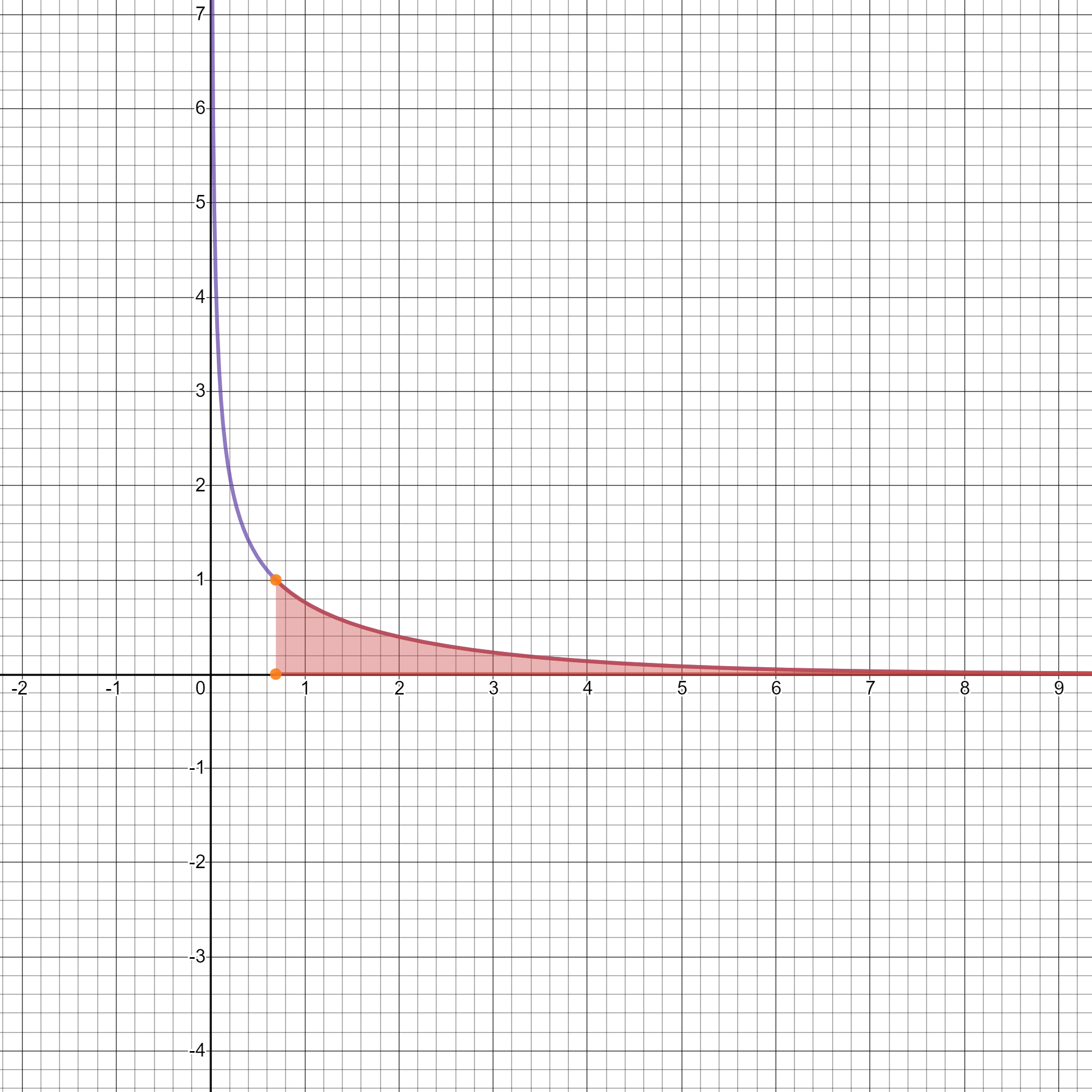# Tag: Definite integrals## Integral of 1/sqrt(e^x-1) from ln(2) to infinity

Hi guys! Hope you are all doing well in these days of quarantine which is hopefully ending soon. I like to spend my time integrating and watching videos about calculus… Read more Integral of 1/sqrt(e^x-1) from ln(2) to infinity## How to solve definite integrals

Indefinite integrals are used to find the formula for the area under a curve of function f(x), whereas definite integrals allow you to calculate the value of the area. It’s like the area of a rectangle, b*h: this is the formula, and in order to find the value you have to plug in the values of b and h. The same is for definite integrals. A definite integral is generally written like this: where a and b are called respectively lower and upper bounds. Also, As you can see, if… Read more How to solve definite integrals

{{#pages}} {{/pages}}
%%footer%%